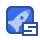# 程序设计基础答案

5星（超过95%的资源）239收藏

〖程序设计基础〗练习题1一、选择题（每题1分，共30分）下列各题A）、B）、C）、D）四个选项中，只有一个选项是正确的，请将正确选项的标记写在题干后的括号内。1.以下的选项中能正确表示Java语言中的一个整型常量的是( )。A) 12. B) -20 C) 1,000 D) 4 5 62.以下选项中，合法的赋值语句是( )。A) a = = 1; B) ++ i; C) a=a + 1= 5; D) y = int ( i );3.若所用变量都已正确定义，以下选项中，非法的表达式是( )。A) a != 4||b==1 B) 'a' % 3 C) 'a' = 1/2 D) 'A' + 324.若有定义int a = 2;则执行完语句a += a -= a * a; 后，a的值是( )。A) 0 B) 4 C) 8 D) -45.设有定义语句int a[]={66,88,99}; 则以下对此语句的叙述错误的是( )。A) 定义了一个名为a的一维数组 B) a数组有3个元素C) a数组的下标为1～3 D)数组中的每个元素是整型6.若a和b均是整型变量并已正确赋值，正确的switch语句是( )。A) switch(a+b)； B) switch( a+b*3.0 ){ ...... } { ...... }C) switch a D) switch ( a%b ){ ...... } { ...... }7.下列语句序列执行后，x 的值是( )。int a=3, b=4, x=5;if( ++aA) 5 B) 3 C) 4 D) 68.下列语句序列执行后，k 的值是( )。int i=6, j=8, k=10, n=5, m=7;if( iA) 9 B) 10 C) 11 D) 129.下列语句序列执行后，r 的值是( )。char ch='8'; int r=10;switch( ch+1 ){ case '7': r=r+3;case '8': r=r+5;case '9': r=r+6; break;default: ;}A) 13 B) 15 C) 16 D) 1010.下列语句序列执行后，j 的值是( )。int j=0;for( int i=3; i>0; i-- ) j+=i;A) 3 B) 4 C) 5 D) 611.下列语句序列执行后，j 的值是( )。int j=9, i=6;while( i-- >3 ) --j;A) 5 B) 6 C) 7 D) 812.下列语句序列执行后，i的值是( )。int i=10;do { i-=2; } while( i>6 );A) 10 B) 8 C) 6 D) 413.为了区分重载多态中同名的不同方法，要求( )。A) 采用不同的形式参数列表 B) 返回值类型不同 C) 调用时用类名或对象名做前缀 D) 参数名不同14.定义主类的类头时可以使用的访问控制符是( )。A) private B) protected C) public D) private protected15.下列整型的最终属性 i 的定义中，正确的是( )。A) static final int i=100; B) final i;C) static int i; D) final float i=1.2f; 16.设 x,y 均为已定义的类名，下列声明对象x1的语句中正确的是( )。A) public x x1= new y( ); B) x x1=x( ); C) x x1=new x( ); D) int x x1;17.下列方法定义中，正确的是( )。A) int x( int a,b ) B) double x( int a,int b){ return (a-b); } { int w; w=a-b; }C) double x( a,b ) D) int x( int a,int b){ return b; } { return a-b; }18.能构成多分支的语句是( )。A) for 语句 B) while 语句 C) switch 语句 D) do -

...展开详情

2014-09-04

• 32
资源
• 2
粉丝
•等级
•分享宗师程序设计基础答案 50积分/C币 立即下载
1/0